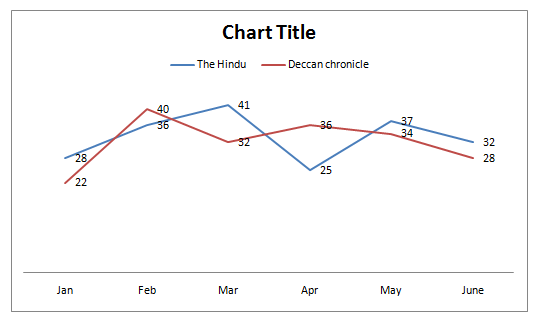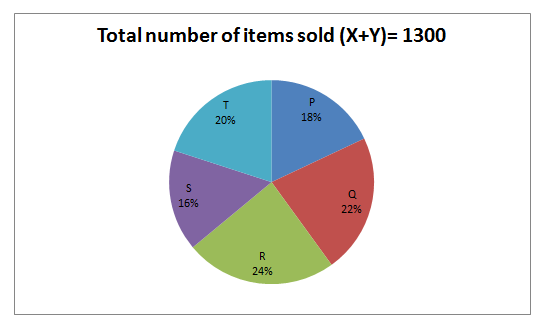# Quantitative Aptitude Questions (Data Interpretation) for SBI Clerk 2018 Day-80

Dear Readers, SBI is conducting Online preliminary Examination for the recruitment of Clerical Cadre. preliminary Examination of SBI Clerk was scheduled from June 2018. To enrich your preparation here we have providing new series of Data Interpretation – Quantitative Aptitude Questions. Candidates those who are appearing in SBI Clerk Prelims Exams can practice these Quantitative Aptitude average questions daily and make your preparation effective.

[WpProQuiz 2168]

Click “Start Quiz” to attend these Questions and view Solutions

Directions (Q. 1 – 5) Study the following information carefully and answer the given questions:

Following line graph shows the total number of readers (In thousands) of two different newspapers in different months in the year 2016.1. Find the difference between the total number of The Hindu readers in the month of Feb, Mar, May and June together to that of Deccan chronicle readers in the month of Jan, Mar, Apr and June together?
1. 32000
2. 41000
3. 28000
4. 24000
5. None of these
1. If the total number of The Hindu and Deccan chronicle readers in April 2017 is increased by 20 % and 25 % respectively while comparing with the previous year April month, then find the sum of total number of The Hindu readers and Deccan chronicle readers in April 2017?
1. 84000
2. 75000
3. 68000
4. 92000
5. None of these
1. If the total number of Deccan chronicle readers in July 2016 is equal to the average number of Deccan chronicle readers in April and May 2016 together and the total number of The Hindu readers in July 2016 is 1/5 of total number of Deccan chronicle readers in July 2016, then find the total number of The Hindu readers in July 2016?
1. 7000
2. 8500
3. 6500
4. 9000
5. None of these
1. Total number of The Hindu readers of all the given months together is approximately what percentage of Deccan chronicle readers of all the given months together?
1. 98 %
2. 86 %
3. 112 %
4. 104 %
5. 121 %
1. Find the ratio between the total number of The Hindu readers in the month of Jan, mar and May together to that of total number of Deccan chronicle readers in the month of Feb, Apr and June together?
1. 51: 55
2. 53: 52
3. 38 : 27
4. 26 : 23
5. None of these

Directions (Q. 6 – 10) Study the following information carefully and answer the given questions:

The following pie chart shows the total no of items sold by five different shops P, Q, R, S and T in a particular month. They sell two different items x and y.1. What is the central angle corresponding to the total number of items sold by shop Q and S together?
1. 127.60
2. 114.40
3. 136.80
4. 142.20
5. None of these
1. If the total number of item X sold by Shop R to that by Shop T is in the ratio of 3 : 4 and the total number of item Y sold by Shop T is 108, then find the total number of item X sold by shop R?
1. 126
2. 114
3. 132
4. 98
5. None of these
1. If the total number of item Y sold by shop Q is 112 and the ratio of item X to Y sold by shop P is 5 : 4, then find the difference between the total number of item X sold by Shop Q to that of total number of item Y sold by Shop P?
1. 86
2. 94
3. 70
4. 62
5. None of these
1. If the total number of items sold by Shop S is 40 % of total number of items purchased by Shop S, then find the total number of unsold items in Shop S?
1. 312
2. 258
3. 376
4. 404
5. None of these
1. Total number of items sold by shop Q and T together is approximately what percentage more/less than the total number of items sold by shop R and S together?
1. 8 % less
2. 12 % more
3. 5 % more
4. 17 % less
5. None of these

Directions (Q. 1 – 5)

The total number of The Hindu readers in the month of Feb, Mar, May and June

= > (36 + 41 + 37 + 32) thousand

= > 146000

The total number of Deccan chronicle readers in the month of Jan, Mar, Apr and June

= > (22 + 32 + 36 + 28) thousand

= > 118000

Required difference = 146000 – 118000 = 28000

The total number of The Hindu readers in April 2017

= > 25*(120/100) = 30 thousand

The total number of Deccan chronicle readers in April 2017

= > 36*(125/100) = 45 thousand

Required sum = 30000 + 45000 = 75000

The total number of Deccan chronicle readers in July 2016

= > (36000 + 34000)/2

= > 35000

The total number of The Hindu readers in July 2016

= > (1/5)*35000

= > 7000

The total number of The Hindu readers in July 2016 = 7000

Total number of The Hindu readers of all the given months together

= > (28 + 36 + 41 + 25 + 37 + 32) thousand

= > 199000

Total number of Deccan chronicle readers of all the given months together

= > (22 + 40 + 32+ 36 + 34+ 28) thousand

= > 192000

Required % = (199000/192000)*100 = 103.64 = 104 %

The total number of The Hindu readers in the month of Jan, mar and May together

= > (28 + 41 + 37) thousand

= > 106000

The total number of Deccan chronicle readers in the month of Feb, Apr and June together

= > (40 + 36 + 28) thousand

= > 104000

Required ratio = 106000: 104000 = 53:52

Directions (Q. 6 – 10)

Central angle of Shop Q and S = [(22 + 16)/100]*360

= > (38/100)*360

= > 136.80

Total number of items sold by Shop R = 1300*(24/100) = 312

Total number of items sold by Shop T = 1300*(20/100) = 260

The ratio of total number of item X sold by Shop R to that by Shop T

= > 3 : 4

The total number of item Y sold by Shop T = 108

The total number of item X sold by Shop T = 260 – 108 = 152

4’s = 152

1’s = 38

The total number of item X sold by Shop R = 3’s = 38*3 = 114

Total number of items sold by Shop Q = 1300*(22/100) = 286

Total number of items sold by Shop P = 1300*(18/100) = 234

The total number of item Y sold by shop Q = 112

The ratio of item X to Y sold by shop P = 5 : 4

9’s = 234

1’s = 26

The total number of item X sold by shop P = 130

The total number of item Y sold by shop P = 104

The total number of item X sold by shop Q = 286 – 112 = 174

Required difference = 174 – 104 = 70

The total number of items sold by Shop S = 1300*(16/100) = 208

208 = (40/100)* the total number of items purchased by Shop S

The total number of items purchased by Shop S

= > (208*100)/40 = 520

Total number of unsold items in Shop S = 520 – 208 = 312

Required % = {[(22 + 20) – (24 + 16)]/(24 + 16)}*100

= > {[42 – 40]/40}*100

= > (2/40)*100 = 5 % more

Daily Practice Test Schedule | Good Luck

 Topic Daily Publishing Time Daily News Papers & Editorials 8.00 AM Current Affairs Quiz 9.00 AM Logical Reasoning 10.00 AM Quantitative Aptitude “20-20” 11.00 AM Vocabulary (Based on The Hindu) 12.00 PM Static GK Quiz 1.00 PM English Language “20-20” 2.00 PM Banking Awareness Quiz 3.00 PM Reasoning Puzzles & Seating 4.00 PM Daily Current Affairs Updates 5.00 PM Data Interpretation / Application Sums (Topic Wise) 6.00 PM Reasoning Ability “20-20” 7.00 PM English Language (New Pattern Questions) 8.00 PM General / Financial Awareness Quiz 9.00 PM